# Equilibrium Analysis of Channel Structure Strategies in Uncertain Environment

## Abstract

In this paper, we consider a pricing decision problem with two competing supply chains which distribute differentiated but competing products in the same market. Each chain can be vertically integrated or decentralized based on the choice of the manufacturer. The manufacturing costs, sales costs and consumer demands are characterized as uncertain variables, whose distributions are estimated by experienced experts. Meanwhile, uncertainty theory and game theory are employed to formulate the pricing decision problems. The equilibrium behaviors (how the supply chain members make their own pricing decisions on wholesale prices and retailer markups) at operational level under three possible scenarios are derived. Numerical experiments are also given to explore the impacts of the parameters’ uncertain degrees on supply chain members’ pricing decisions. The results demonstrate that the supply chain uncertain factors have great influences on equilibrium prices. In addition, we also evaluate the effects of competing intensity (substitutability) of the two products on the strategy behaviors, vertically integrated channel strategy versus decentralized strategy, of the manufacturers. It is found that the manufacturers are better off to distribute their products through a decentralized channel rather than an integrated one when the substitutability is greater than some value. Besides, the uncertain factors in the supply chain might reduce the value contrast to the one in deterministic case. Some other interesting managerial highlights are also provided in this paper.

## Introduction

In this paper, we investigate a pricing competition problem in some special competing supply chains in which differentiated but substitutable products are sold into the same market. These competing supply chains often consist of only a few upstream manufacturers, each of which distributes its products through exclusive downstream outlets who usually carry only one product line. This exclusive dealership is not uncommon in industries like petrol, automobiles, some electronic products, softdrinks, fastfoods, and so on. For instance, a petrol gas station often retails gasolines from some certain oil producer, and a 4S store usually carries some certain car brand or cars from a specific manufacturer. For convenience, we use “manufacturer” to represent the upstream firm and “retailer” to the downstream channel participant in the following discussion.

### Motivation

Nowadays, hi-tech products, e.g., digital devices, are often updated quickly. The demands and costs of these products, especially of new products, are usually with no historical data. Even though sometimes the historical data may be available, it may not be applicable due to the highly changeable markets. In these cases, we have to rely on belief degrees given by experienced managers and experts. Surveys have indicated, however, that human beings usually estimate a much wider range of values than they actually take. Therefore, human belief degree should not be treated as random variable or fuzzy variable. When some indeterminate phenomena, expressed by human language like “approximately 4000” or “high costs”, behave neither randomness nor fuzziness, uncertainty theory, initiated by Liu  and refined by Liu  based on normality, duality, subadditivity, and product axioms, is a legitimate approach to dealing with circumstances where only belief degree is available.

This paper focuses on the pricing decisions of the two substitutable products distributed by two totally separate supply chains. More specially, the products’ demands and costs are characterized as uncertain variables whose distributions are estimated by belief degrees. How should the supply chain members make their own pricing decisions on wholesale prices and retailer markups with uncertain demands and costs? What effects might the parameters’ uncertain degrees (decided by the available information and experts’ preference) have on supply chain members’ pricing decisions? How do uncertainties and competing intensity (substitutability) between the two products affect the duopoly manufacturers’ strategy behaviors, in vertically integrated channel structures versus decentralized structures?

In order to cover these problems, uncertainty-theory-based and game-theory-based models are employed to derive the optimal equilibria in different scenarios. Numerical experiments are also given to explore the effects and strategies.

### Literature Review

By now, considerable attentions have been focused on the pricing competition in totally separate chains as mentioned both from scholars and practitioners. McGuire and Staelin  initiated the research and investigated the effects of the substitutability on the structure strategies in duopoly supply chains where each manufacturer distributes its goods through a single exclusive retailer. Coughlan  tested results that integration of the marketing functions results in greater pricing competition and lower prices than the use of independent marketing middlemen by survey data from the international semiconductor industry. Recently, Anderson and Bao  extended the model of Coughlan  from two entirely separate chains to a more general context with arbitrary competing supply chains, and also demonstrated that the underlying market shares play a very important role on the equilibrium behaviors. Li and Li  studied the two sustainable supply chains under competition in product sustainability under different structures.

The work above has typically focused on deterministic demands and costs. In fact, the real world has many indeterminate factors which cannot be ignored when making pricing decisions. Those indeterminacies, such as material costs, customer incomes, workers’ expenses and technology improvements, usually affect the manufacturing costs and consumer demands. Some of them can be described as random variables if we can attain accurate distributions. Therefore, Xiao and Yang  studied a price and service competition of two supply chains with risk-averse retailers under stochastic demand. They also analyzed the impacts of the retailer’s risk sensitivity on the manufacturers’ equilibrium strategies. Wu et al.  considered a joint pricing and quantity competition between two separate supply chains in random environment and explored the effect of randomness on the equilibrium behaviors of the supply chain members. Shi et al.  utilized a game-theory-based framework to formulate the power in a supply chain and examined how power structure and demand indeterminacy affect supply chain members’ performances. Mahmoodi and Eshghi  studied this pricing problem in duopoly supply chains with stochastic demand and explored the effect of competition and demand indeterminacy intensity on the equilibrium of the structures by a numerical example.

In addition, the others can be described as fuzzy variables if we cannot estimate the accurate distributions due to the complicated and changeable environments. Fuzzy set theory has been introduced to the pricing decision game recently. Zhou et al.  considered the pricing decision problem in supply chains composed of a manufacturer and a retailer under fuzzy environment. Zhao et al. [12, 13] studied the pricing problem of two substitutable products in supply chains with different structures, in which the consumer demands and manufacturing costs are described by fuzziness. Following that, Zhao et al.  added the manufacturer service to the pricing problem in a two-echelon fuzzy supply chain, in which two competitive manufacturers supply two substitutable products to one common retailer. Liu and Xu  and Ke et al.  studied the pricing problem of one product in a fuzzy supply chain consisting of one manufacturer and two competitive retailers.

To the best of our knowledge, there are little researches on the supply chain pricing decision problems with indeterminate factors behaving neither randomness nor fuzziness. Differing from the literature above, this paper addresses the pricing equilibrium under circumstances where only belief degree is available by applying uncertainty theory. Nowadays, uncertainty theory has been well developed in many aspects, such as uncertain set , uncertain differential Eq. , uncertain sequence , etc. Besides, the new theory has been successfully applied to deal with many uncertain decision-making problems, e.g., option pricing [20, 21], portfolio selection , facility location , differential games , project scheduling problem , supply chain pricing problem [30, 31] and network problem . Specially, Huang and Ke  studied a pricing decision problem in supply chain with duopoly manufacturers and a common retailer, and explored the pricing decisions with three different power structures under uncertain environment by applying uncertainty theory and game theory. Different from the literature above, this paper considers a pricing problem in two totally separate supply chains and focuses on pricing decisions with uncertainty at both operational level and strategy level.

For simplicity of analysis, we restrict our research on a pricing problem between two manufacturers, each of which distributes its product through a single exclusive retailer. The manufacturers can choose integrated strategy of distributing their products through a company store (owned by the manufacturer) or through an independent retail outlet (privately owned). The manufacturers are often much larger than the retailers, hence the retailers have little power on the wholesale prices. If the manufacturer chooses an independent retailer, it may lose control of the sales price. Consequently, the manufacturers with dominant power choose the wholesale prices while the retailers decide the retail prices by adding some margins (markup prices). Specifically, the manufacturing costs, sales costs and demands are characterized as uncertain variables whose distributions are estimated by experienced managers’ or marketing experts’ belief degrees. Meanwhile, Stackelberg and Bertrand models are employed to formulate the pricing decision problems at operational level. We then derive the equilibrium prices and profits in the three possible structures from the models. Numerical experiments are also provided to illustrate the effects of the uncertain degrees of the parameters on the supply chain members’ pricing decisions and strategy behaviors.

The remainder of this paper is organized as follows: Introductions of uncertainty theory and uncertain programming model are presented in Section “Preliminaries”. Following that, some useful notations and necessary assumptions are discussed in Section “Problem Description”. Three models are employed to derive the equilibria under three possible scenarios in Section “Models and Solution Approaches”. Afterwards, in Section “Strategy Decision Analysis”, numerical experiments are applied to demonstrate the effectiveness of the models and then examine the impacts of uncertain degrees and competing intensity on equilibrium behaviors both at operational level and strategy level. Some management highlights and conclusions are discussed in “Conclusions” section.

## Preliminaries

In this section, we will introduce some important concepts and theorems of uncertainty theory for modeling the pricing decision problem with human belief degree. Let Γ be a nonempty set and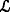a σ-algebra over Γ. Each element Λ inis called an event.

### Definition 1

Liu  The set function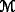is called an uncertain measure if it satisfies:

### Axiom 1

(Normality Axiom)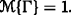.

### Axiom 2

(Duality Axiom)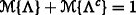for any event Λ.

### Axiom 3

(Subadditivity Axiom) For every countable sequence of events {Λ i }, i=1,2,, we have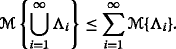Besides, the product uncertain measure on the product σ-algebrawas defined by Liu  as follows:

### Axiom 4

(Product Axiom) Let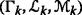be uncertainty spaces for k=1,2, The product uncertain measureis an uncertain measure satisfying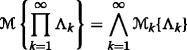where Λ k are arbitrarily chosen events from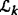for k=1,2,, respectively.

### Definition 2

Liu [ 1 ] An uncertain variable is a measurable function ξ from an uncertainty space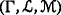to the set of real numbers, i.e., for any Borel set B of real numbers, the set

$$\{\xi\in B\}=\{\gamma\in \Gamma \ \big|\ \xi(\gamma)\in B\}$$

is an event.

### Definition 3

Liu [ 34 ] The uncertain variables ξ 1,ξ 2,,ξ n are said to be independent if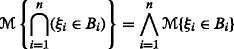for any Borel sets B 1,B 2,,B n .

Sometimes, we should know uncertainty distribution to model real-life uncertain optimization problems.

### Definition 4

Liu [ 1 ] The uncertainty distribution Φ of an uncertain variable ξ is defined by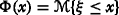for any real number x.

An uncertainty distribution Φ is referred to be regular if its inverse function Φ −1(α) exists and is unique for each α[0,1].

### Lemma 1

Liu [ 2 ] Let ξ 1,ξ 2,,ξ n be independent uncertain variables with regular uncertainty distributions Φ 1,Φ 2,,Φ n , respectively. If the function f(x 1,x 2,,x n ) is strictly increasing with respect to x 1,x 2,,x m and strictly decreasing with respect to x m+1,x m+2,,x n , then

$$\xi=f(\xi_{1}, \xi_{2}, \cdots, \xi_{n})$$

is an uncertain variable with inverse uncertainty distribution

$$\Phi^{-1}(\alpha)=f(\Phi_{1}^{-1}(\alpha),\cdots,\Phi_{m}^{-1}(\alpha),\Phi_{m+1}^{-1}(1-\alpha),\cdots,\Phi_{n}^{-1}(1-\alpha)).$$

### Definition 5

Liu [ 1 ] Let ξ be an uncertain variable. The expected value of ξ is defined by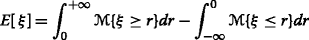provided that at least one of the above two integrals is finite.

### Lemma 2

Liu [ 2 ] Let ξ be an uncertain variable with uncertainty distribution Φ. If the expected value exists, then

$$E[\xi]=\int_{0}^{+\infty} (1-\Phi(x))d x-\int_{-\infty}^{0} \Phi(x)d x.$$

### Lemma 3

Liu [ 2 ] Let ξ be an uncertain variable with regular uncertainty distribution Φ. If the expected value exists, then

$$E[\xi]={\int_{0}^{1}} \Phi^{-1}(\alpha)d \alpha.$$

### Example 1

Let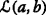be a linear uncertain variable, then

$$\begin{array}{@{}rcl@{}} \Phi(x)= \left\{\begin{array}{ll} \quad \quad 0,\ &x<a\\ (x-a)/(b-a), \ &a\le x\le b\\ \quad \quad 1, \ &x>b \end{array} \right. \end{array}$$
(1)

and its inverse uncertainty distribution is Φ −1(α)=a+(ba)α. The expected value can be attained

\begin{aligned} E[\xi]=&{\int_{0}^{1}}(a+(b-a)\alpha) \mathrm{d}\alpha=\frac{a+b}{2}. \end{aligned}
(2)

### Example 2

The zigzag uncertain variable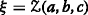has an uncertainty distribution

$$\begin{array}{@{}rcl@{}} \Phi(x)= \left\{\begin{array}{ll} \quad \quad \quad 0,\ & x< a\\ \quad (x-a)/2(b-a),\ & a\le x\le b\\ (x+c-2b)/ 2(c-b),\ & b< x\le c\\ \quad \quad \quad 1, \ & x> c \end{array} \right. \end{array}$$
(3)

and its inverse uncertainty distribution is

$$\begin{array}{@{}rcl@{}} \Phi^{-1}(\alpha)= \left\{\begin{array}{ll} (1-2\alpha)a+2\alpha b,& \alpha <0.5 \\ (2-2\alpha)b+(2\alpha-1)c, \ & \alpha \ge 0.5. \end{array} \right. \end{array}$$
(4)

Thus, its expected value is as follows:

\begin{aligned} E[\xi]=&\int_{0}^{0.5}((1-2\alpha)a + 2\alpha b)\mathrm{d}\alpha+\int_{0.5}^{1}((2-2\alpha)b + (1-2\alpha) c)\mathrm{d}\alpha=\frac{a+2b+c}{4}. \end{aligned}
(5)

### Lemma 4

Liu [ 35 ] Let ξ 1,ξ 2,,ξ n be independent uncertain variables with regular uncertainty distributions Φ 1,Φ 2,,Φ n , respectively. A function f(x 1,x 2,,x n ) is strictly increasing with respect to x 1,x 2,,x m and strictly decreasing with respect to x m+1,x m+2,,x n . Then, the expected value of ξ=f(ξ 1,ξ 2,,ξ n ) is

\begin{aligned} \text{E}[\xi]={\int_{0}^{1}} f(\Phi_{1}^{-1}(\alpha),\cdots,\Phi_{m}^{-1}(\alpha),\Phi_{m+1}^{-1}(1-\alpha),\cdots,\Phi_{n}^{-1}(1-\alpha))\mathrm{d}\alpha \end{aligned}
(6)

provided that the expected value E[ξ] exists.

### Example 3

Let ξ and η be two positive independent uncertain variables with regular uncertainty distributions Φ and Ψ, respectively. Then, we have

\begin{aligned} E\left[\frac{\xi}{\eta}\right]={\int_{0}^{1}}\frac{\Phi^{-1}(\alpha)}{\Psi^{-1}(1-\alpha)}\mathrm{d}\alpha. \end{aligned}
(7)

Uncertain programming, as a type of mathematical programming involving uncertain variables, was initiated by Liu . The general form of uncertain programming is shown as follows: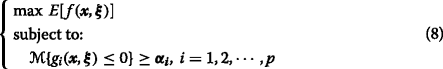where x is a decision vector, ξ is an uncertain vector of parameters, E[f(x,ξ)] means the expected value of the objective function while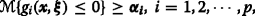is a set of chance constraints.

With the above concepts and lemmas, we can model the pricing decision problem in uncertain environments.

## Problem Description

We restrict our research on two entirely separate supply chains which distribute differentiated but competing products to the same market. The channel structures are either vertically integrated or decentralized based on the strategies of the manufacturers. As shown in Fig. 1, each manufacturer can employ one retailer per market area to retail its products (Decentralized Strategy); or it can distribute its products to consumers directly using its own company store (Integrated Strategy). It is assumed that the manufacturers perform as a Stackelberg leader in each chain (in the case of decentralized strategy) but there is no dominant power between the two supply chains.

The ith manufacturer (M 1 or M 2) produces product i at unit cost c i and wholesales the product to a specified retailer R i , i=1,2. Then the retailer sells the product to consumers with unit sales cost s i . The manufacturer chooses the wholesale price w i while the retailer decides the sales price p i =w i +r i by adding some margin (markup price r i ) to the wholesale price. The consumers are price sensitive and the demand functions are described as follows:

$$q_{i}=d_{i}-\delta p_{i}+\gamma \sum_{j=1,j\not=i}^{n}(p_{j}-p_{i}),\quad i=1,2,\cdots,n.$$

In this paper, we merely explore two separate supply chains, and then the demand function can be simplified as follows:

$$q_{i}=d_{i}-(\delta+\gamma) p_{i}+\gamma p_{3-i},\quad i=1,2$$

where d i is the market base, δ denotes the price-sensitivity of the consumers, γ is the substitutability of the two products and p i is the sales price of product i. Note that changes in d i alter the relative product preferences. Let β=δ+γ. The above function can be rewritten as

$$q_{i}=d_{i}-\beta p_{i}+\gamma p_{3-i},\quad i=1,2.$$

This linear demand function is widely applied in supply chain management [5 , 37 , 38]. Due to the complicated and changeable environment, the price elastic coefficient cannot be estimated precisely. In many instances, $$\tilde {\beta }$$ and $$\tilde {\gamma }$$ can be characterized as uncertain variables.

The notations are listed as follows:

• unit manufacturing cost of product i

• unit sales cost of product i

• unit wholesale price of product i

• unit markup price of product i

• unit retail price of product i, where p i =w i +r i

• market base of product i

• demand of product i

• profit of manufacturer i:$$\pi _{m_{i}}=(w_{i}-\tilde {c}_{i})q_{i},\ i= 1, 2$$

• profit of retailer i:$$\pi _{r_{i}}=(r_{i}-\tilde {s}_{i})q_{i},\ i= 1, 2$$

### Assumption 1

Because the product demand should be more sensitive to changes of its price than to changes of the other product, it is assumed that the elastic coefficients $$\tilde {\beta }$$ and $$\tilde {\gamma }$$ satisfy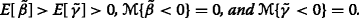### Assumption 2

All the uncertain coefficients are assumed nonnegative and mutually independent.

### Assumption 3

(Full information) The manufacturers and retailers have same information and estimations on the demands and the costs of other channel members.

### Assumption 4

(Risk neutral) It is assumed that all the channel members are risk neutral and desire to maximize the expected profits.

Because the costs cannot exceed the retail price and markup, and the demands are always positive, then we have the following assumption.

### Assumption 5

(Positive assumption) It is assumed that the costs cannot exceed the retail price and markup, and the demands are always positive, shown as follows: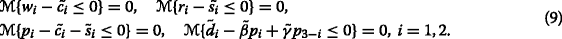Let $$\tilde {c}_{i}, \tilde {s}_{i},\tilde {d}_{i}, \tilde {\beta }$$ and $$\tilde {\gamma }$$ be positive independent uncertain variables with regular uncertainty distributions $$\Phi _{c_{i}}$$, $$\Phi _{s_{i}},\Phi _{d_{i}}$$, Φ β , and Φ γ . For simplicity, we define

$$\begin{array}{ll} E[\tilde{a}^{\alpha} \tilde{b}^{\alpha}]={\int_{0}^{1}}\Phi_{a}^{-1}(\alpha)\Phi_{b}^{-1}(\alpha)\mathrm{d} \alpha,\quad E[\tilde{a}^{1-\alpha} \tilde{b}^{1-\alpha}]={\int_{0}^{1}}\Phi_{a}^{-1}(1-\alpha)\Phi_{b}^{-1}(1-\alpha)\mathrm{d} \alpha,\\[0.2cm] E[\tilde{a}^{\alpha} \tilde{b}^{1-\alpha}]={\int_{0}^{1}}\Phi_{a}^{-1}(\alpha)\Phi_{b}^{-1}(1-\alpha)\mathrm{d} \alpha,\quad E[\tilde{a}^{1-\alpha} \tilde{b}^{\alpha}]={\int_{0}^{1}}\Phi_{a}^{-1}(1-\alpha)\Phi_{b}^{-1}(\alpha)\mathrm{d} \alpha\\ \end{array}$$
(8)

where $$\Phi _{a}^{-1}$$ and $$\Phi _{b}^{-1}$$ are the inverse uncertainty distributions of uncertain variables $$\tilde {a}$$ and $$\tilde {b}$$, respectively.

### Proposition 1

If Assumption 5 holds, the expected profits of the participants can be transformed as follows:

{} \begin{aligned} \pi_{m_{i}} =&-\text{E}[\tilde{\beta}] {w_{i}^{2}}+\text{E}[\tilde{\gamma}] w_{3-i}w_{i}+(-\text{E}[\tilde{\beta}] r_{i}+ \text{E}[\tilde{\gamma}] r_{3-i}+E[\tilde{d}_{i}]+E[\tilde{c}_{i}^{1-\alpha}\tilde{\beta}^{1-\alpha}]) w_{i}\\ \ & -E[\tilde{c}_{i}^{1-\alpha} \tilde{d_{i}}^{\alpha}]+E[\tilde{c}_{i}^{1-\alpha} \tilde{\beta}^{1-\alpha}] r_{i} - E[\tilde{c_{i}}^{1-\alpha} \tilde{\gamma}^{\alpha}](r_{3-i}+w_{3-i}), \\ \pi_{r_{i}} =&-E[\tilde{\beta}] {r_{i}^{2}}+E[\tilde{\gamma}]r_{3-i}r_{i}+(-E[\tilde{\beta}] w_{i}+ E[\tilde{\gamma}] w_{3-i}+E[\tilde{d}_{i}]+E[\tilde{s}_{i}^{1-\alpha}\tilde{\beta}^{1-\alpha}]) r_{i}\\ \ & -E[\tilde{s}_{i}^{1-\alpha} \tilde{d}_{i}^{\alpha}]+E[\tilde{s}_{i}^{1-\alpha} \tilde{\beta}^{1-\alpha}] w_{i} - E[\tilde{s}_{i}^{1-\alpha} \tilde{\gamma}^{\alpha}](r_{3-i}+w_{3-i}),\ i=1,2. \end{aligned}
(9)

### Proof1.

Let $$\pi _{m_{i}}=E[(w_{i}-\tilde {c}_{i})(\tilde {d}_{i}-\tilde {\beta } (r_{i}+w_{i})+ \tilde {\gamma } (r_{3-i}+w_{3-i}))],\ i=1,2.$$ Obviously, if the conditions in Assumption 5 hold, $$\pi _{m_{i}}$$ is monotone increasing with $$\tilde {d}_{i},\tilde {\gamma }$$ and monotone decreasing with $$\tilde {c}_{i},\tilde {\beta }$$. Referring to Lemma 4, the expected function is

{} \begin{aligned} \pi_{m_{i}} =&{\int_{0}^{1}}[\!(w_{i}-\Phi_{c_{i}}^{-1}(1-\alpha))(\Phi_{d_{i}}^{-1}(\alpha)\!-\Phi_{\beta}^{-1}(1-\alpha) (r_{i}+w_{i})+\Phi_{\gamma}^{-1}(\alpha)(r_{3-i}+w_{3-i}))]d \alpha\\ =&{\int_{0}^{1}}[(w_{i}-c_{i}^{1-\alpha})(\tilde{d_{i}}^{\alpha}-\beta^{1-\alpha} (r_{i}+w_{i})+\tilde {\gamma}^{\alpha}(r_{3-i}+w_{3-i}))]d\alpha.\\ \end{aligned}
(10)

Then

{} \begin{aligned} \pi_{m_{i}} =&-\text{E}[\tilde{\beta}] {w_{i}^{2}}+\text{E}[\tilde{\gamma}] w_{3-i}w_{i}+(-\text{E}[\tilde{\beta}] r_{i}+ \text{E}[\tilde{\gamma}] r_{3-i}+E[\tilde{d}_{i}]+E[\tilde{c}_{i}^{1-\alpha}\tilde{\beta}^{1-\alpha}]) w_{i}\\ \ & -E[\tilde{c_{i}}^{1-\alpha} \tilde{d}_{i}^{\alpha}]+E[\tilde{c}_{i}^{1-\alpha} \tilde{\beta}^{1-\alpha}] r_{i} - E[\tilde{c_{i}}^{1-\alpha} \tilde{\gamma}^{\alpha}](r_{3-i}+w_{3-i}). \\ \end{aligned}
(11)

In the same way, we can get the crisp forms of the expected profit functions $$\pi _{r_{i}}$$ in Proposition 1, i=1,2.

## Models and Solution Approaches

In this section, the uncertain programming models based on Stackelberg and Nash game theory are employed to derive the equilibrium prices in different channel structures.

### Decentralized Structure (DD)

In the first case, both the chains are decentralized and the competition becomes a four-members game. The detailed decision sequence is as follows: The two Stackelberg manufacturers simultaneously announce their wholesale prices w i to maximize their own profits allowing for the retailers’ optimal responses. Then, the two retailers, performing as followers, non-cooperatively choose the markup pricing schemes or unit sales commission r i , respectively, to maximize their own profits conditional on the other retailer’s decision. Then, the retail prices are decided as p i =w i +r i ,i=1,2, as well as the sales quantities. It is assumed that the manufacturers and retailers are risk neutral and desire to maximize their expected profits.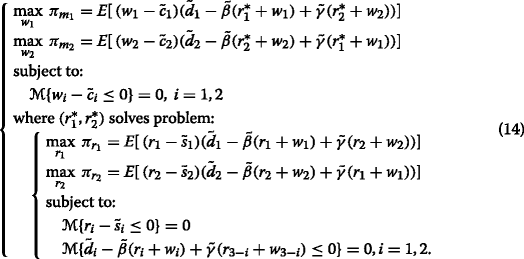To solve this Nash-Stackelberg-Nash game model, opposite to the decision sequence, we should derive the Nash equilibrium in the lower level for the given wholesale prices w 1 and w 2 specified by the manufacturers in advance.

Referring to the expected value functions of the retailers with the given wholesale prices, we can get

\begin{aligned} \frac {\partial^{2}\pi_{r_{1}}(r_{1},r_{2})}{\partial {r_{1}^{2}}}=-2E[\tilde{\beta}]<0,\quad \frac {\partial^{2}\pi_{r_{2}}(r_{1},r_{2})}{\partial {r_{2}^{2}}}=-2E[\tilde{\beta}]<0, \end{aligned}
(12)

with the assumption that $$E[\tilde {\beta }] >E[\tilde {\gamma }]>0$$. Hence, $$\pi _{r_{1}}$$ and $$\pi _{r_{2}}$$ are concave in r 1 and r 2, respectively. Then we can get the Nash equilibrium by setting the first-order derivatives equaling 0 as follows:

\begin{aligned} \frac {\partial \pi_{r_{1}}}{\partial r_{1}}=&-2E[\tilde{\beta}] r_{1}+E[\tilde{\gamma}]r_{2}-E[\tilde{\beta}] w_{1}+ E[\tilde{\gamma}] w_{2}+E[\tilde{d}_{1}]+E[\tilde{s}_{1}^{1-\alpha}\tilde{\beta}^{1-\alpha}]=0, \\ \frac {\partial \pi_{r_{2}}}{\partial r_{2}}=&-2E[\tilde{\beta}] r_{2}+E[\tilde{\gamma}]r_{1}-E[\tilde{\beta}] w_{2}+ E[\tilde{\gamma}] w_{1}+E[\tilde{d}_{2}]+E[\tilde{s}_{2}^{1-\alpha}\tilde{\beta}^{1-\alpha}]=0. \end{aligned}
(13)

The followers’ optimal responses to (w 1,w 2) can be easily obtained by solving the above two equations as follows:

\begin{aligned} r_{1}^{*}(w_{1},w_{2})=&\frac{-2E[\tilde{\beta}]^{2}+E[\tilde{\gamma}]^{2}}{4E[\tilde{\beta}]^{2}-E[\tilde{\gamma}]^{2}}w_{1}+\frac{E[\tilde{\beta}]E[\tilde{\gamma}] }{4E[\tilde{\beta}]^{2}-E[\tilde{\gamma}]^{2}}w_{2}+ \\&\frac{2E[\tilde{\beta}](E[\tilde{d}_{1}]+ E[\tilde{\beta}^{1-\alpha}\tilde{s}_{1}^{1-\alpha}])+E[\tilde{\gamma}](E[\tilde{d}_{2}]+E[\tilde{\beta}^{1-\alpha}\tilde{s}_{2}^{1-\alpha}])}{4E[\tilde{\beta}]^{2}-E[\tilde{\gamma}]^{2}},\\ \indent r_{2}^{*}(w_{1},w_{2})=&\frac{-2E[\tilde{\beta}]^{2}+E[\tilde{\gamma}]^{2}}{4E[\tilde{\beta}]^{2}-E[\tilde{\gamma}]^{2}}w_{2}+\frac{E[\tilde{\beta}]E[\tilde{\gamma}] }{4E[\tilde{\beta}]^{2}-E[\tilde{\gamma}]^{2}}w_{1}+\\& \frac{2E[\tilde{\beta}](E[\tilde{d}_{2}]+ E[\tilde{\beta}^{1-\alpha}\tilde{s}_{2}^{1-\alpha}])+E[\tilde{\gamma}](E[\tilde{d}_{1}]+E[\tilde{\beta}^{1-\alpha}\tilde{s}_{1}^{1-\alpha}])}{4E[\tilde{\beta}]^{2}-E[\tilde{\gamma}]^{2}}.\\ \end{aligned}
(14)

Given the two retailers’ optimal responses, substituting Eq. (14) into the profit functions of the manufacturers, we obtain

\begin{aligned} \pi_{m_{i}}&=E\left[(w_{i}-\tilde{c}_{i})(\tilde{d}_{i}-\tilde{\beta}(r_{i}^{*}+w_{i})+\tilde{\gamma}(r_{3-i}^{*}+w_{3-i}))\right]\\ &=E\left[(w_{i}-\tilde{c}_{i})\left(\tilde{d}_{i}-\tilde{\beta}\left(\frac{2E[\tilde{\beta}]^{2}}{4E[\tilde{\beta}]^{2} -E[\tilde{\gamma}]^{2}} w_{i}+\frac{E[\tilde{\beta}]E[\tilde{\gamma}]}{4E[\tilde{\beta}]^{2} -E[\tilde{\gamma}]^{2}}w_{3-i}+S_{i}\right.\right)\right.\\ &\qquad\left.\left.+\tilde{\gamma}\left(\frac{2E[\tilde{\beta}]^{2}}{4E[\tilde{\beta}]^{2} -E[\tilde{\gamma}]^{2}} w_{3-i}+\frac{E[\tilde{\beta}]E[\tilde{\gamma}]}{4E[\tilde{\beta}]^{2} -E[\tilde{\gamma}]^{2}}w_{i}+S_{3-i}\right)\right)\right]\\ &=E[\tilde{d}_{i}]w_{i}-\frac{2E[\tilde{\beta}]^{3}}{4E[\tilde{\beta}]^{2} -E[\tilde{\gamma}]^{2}} {w_{i}^{2}}-\frac{E[\tilde{\beta}]^{2}E[\tilde{\gamma}]}{4E[\tilde{\beta}]^{2} -E[\tilde{\gamma}]^{2}}w_{3-i}w_{i}-E[\tilde{\beta}]S_{i}w_{i}\\ &\qquad+\frac{2E[\tilde{\beta}]^{2}E[\tilde{\gamma}]}{4E[\tilde{\beta}]^{2} -E[\tilde{\gamma}]^{2}} w_{3-i}w_{i}+\frac{E[\tilde{\beta}]E[\tilde{\gamma}^{2}]}{4E[\tilde{\beta}]^{2} -E[\tilde{\gamma}]^{2}}{w_{i}^{2}}+S_{3-i}w_{i}\\ &\qquad\!-E[\tilde{c}_{i}^{1-\alpha}\tilde{d}_{i}^{\alpha}]\,+\,E[\tilde{c}_{i}^{1-\alpha}\tilde{\beta}^{1-\alpha}]\left(\frac{2E[\tilde{\beta}]^{2}}{4E[\tilde{\beta}]^{2} \,-\,E[\tilde{\gamma}]^{2}} w_{i}\,+\,\frac{E[\tilde{\beta}]E[\tilde{\gamma}]}{4E[\tilde{\beta}]^{2\!} -\!E[\tilde{\gamma}]^{2}}w_{3-i}\,+\,S_{i}\right)\\ &\qquad-E[\tilde{c}_{i}^{1-\alpha}\tilde{\gamma}^{\alpha}]\left(\frac{2E[\tilde{\beta}]^{2}}{4E[\tilde{\beta}]^{2} -E[\tilde{\gamma}]^{2}} w_{3-i}+\frac{E[\tilde{\beta}]E[\tilde{\gamma}]}{4E[\tilde{\beta}]^{2} -E[\tilde{\gamma}]^{2}}w_{i}+S_{3-i}\right) \end{aligned}
(15)

where

\begin{aligned} \indent &S_{i}=\frac{2E[\tilde{\beta}](E[\tilde{d}_{i}]+ E[\tilde{\beta}^{1-\alpha}\tilde{s}_{i}^{1-\alpha}])+E[\tilde{\gamma}](E[\tilde{d}_{3-i}]+E[\tilde{\beta}^{1-\alpha}\tilde{s}_{3-i}^{1-\alpha}])}{4E[\tilde{\beta}]^{2}-E[\tilde{\gamma}]^{2}},\ i=1,2.\\ \end{aligned}

Referring to Eq. (15), we can obtain the second-order derivatives of the equivalent objective functions $$\pi _{m_{i}}$$ as follows:

\begin{aligned} \frac {\partial^{2}\pi_{m_{i}}}{\partial {w_{i}^{2}}}=\frac{-2E[\tilde{\beta}](2E[\tilde{\beta}]^{2}-E[\tilde{\gamma}]^{2})}{4E[\tilde{\beta}]^{2}-E[\tilde{\gamma}]^{2}}<0,\ i=1,2. \end{aligned}

Hence, by differentiating $$\pi _{m_{i}}$$ and equating the expressions to 0, we have

\begin{aligned} \frac {\partial\pi_{m_{1}}(w_{1},w_{2})}{\partial w_{1}}=-2Aw_{1}+Bw_{2}+E[\tilde{d}_{1}]-E[\tilde{\beta}]S_{1}+E[\tilde{\gamma}]S_{2}+C_{1}=0,\\ \frac {\partial\pi_{m_{2}}(w_{1},w_{2})}{\partial w_{2}}=-2Aw_{2}+Bw_{1}+E[\tilde{d}_{2}]-E[\tilde{\beta}]S_{2}+E[\tilde{\gamma}]S_{1}+C_{2}=0, \end{aligned}

and the leaders’ equilibrium prices (w 1,w 2) can be easily obtained by solving the above two equations

\begin{aligned} \indent &w_{1}^{*}= \frac{2A(E[\tilde{d}_{1}]-E[\tilde{\beta}]S_{1}+E[\tilde{\gamma}]S_{2}+C_{1})+B(E[\tilde{d}_{2}]-E[\tilde{\beta}]S_{2}+E[\tilde{\gamma}]S_{1}+C_{2})} {4 A^{2}-B^{2}},\\ \indent &w_{2}^{*}=\frac {2A(E[\tilde{d}_{2}]-E[\tilde{\beta}]S_{2}+E[\tilde{\gamma}]S_{1}+C_{2})+B(E[\tilde{d}_{1}]-E[\tilde{\beta}]S_{1}+E[\tilde{\gamma}]S_{2}+C_{1})} {4A^{2}-B^{2}} \end{aligned}
(16)

where

\begin{array}{@{}rcl@{}} \begin{aligned} \indent &A=\frac{2E[\tilde{\beta}]^{3}-E[\tilde{\beta}]E[\tilde{\gamma}]^{2}}{4E[\tilde{\beta}]^{2}-E[ \tilde{\gamma}]^{2}}, \indent B=\frac{E[\tilde{\beta}]^{2}E[\tilde{\gamma}]}{4E[\tilde{\beta}]^{2}-E[ \tilde{\gamma}]^{2}},\\ &C_{i}=\frac{2E[\tilde{\beta}]^{2}E[\tilde{c}_{i}^{1-\alpha}\tilde{\beta}^{1-\alpha}]+E[\tilde{\beta}]E[\tilde{\gamma}] E[\tilde{c_{i}^{1-\alpha}}\tilde{\gamma}^{\alpha}]}{4E[\tilde{\beta}]^{2}-E[\tilde{\gamma}]^{2}},\ i=1,2. \end{aligned} \end{array}

Then, we can get the equilibrium prices of the two retailers as follows:

{} {\begin{aligned} r_{1}^{*}=\frac{(-2E[\tilde{\beta}]^{2}+E[\tilde{\gamma}]^{2})w_{1}^{*}+E[\tilde{\beta}]E[\tilde{\gamma}] w_{2}^{*}+2E[\tilde{\beta}](E[\tilde{d}_{1}]+ E[\tilde{\beta}\tilde{s}_{1}])+E[\tilde{\gamma}](E[\tilde{d}_{2}]+E[\tilde{\beta}\tilde{s}_{2}])}{4E[\tilde{\beta}]^{2}-E[\tilde{\gamma}]^{2}},\\ \indent r_{2}^{*}=\frac{(-2E[\tilde{\beta}]^{2}+E[\tilde{\gamma}]^{2})w_{2}^{*}+E[\tilde{\beta}]E[\tilde{\gamma}] w_{1}^{*}+2E[\tilde{\beta}](E[\tilde{d}_{2}]+ E[\tilde{\beta}\tilde{s}_{2}])+E[\tilde{\gamma}](E[\tilde{d}_{1}]+E[\tilde{\beta}\tilde{s}_{1}])}{4E[\tilde{\beta}]^{2}-E[\tilde{\gamma}]^{2}}. \end{aligned}}

### Mixed Structures (DI and ID)

The second possible structure is that one chain is vertically integrated and the other chain is decentralized. The integrated one can be seen as a manufacturer who retails its product directly or a retailer who purchases products from its own factory (the wholesale price is the manufacturing cost).

In this scenario, the number of the competitors reduces to three, stated as independent manufacturer (M1), independent retailer (R1) and integrated “manufacturer” (or integrated “retailer”) (M2). The detailed decision sequence is as follows: the integrated “manufacturer” and the independent manufacturer simultaneously announce the retail or wholesale prices to maximize their own profits conditional on the retailer’s response. Then the retailer chooses the most profitable markup. It is assumed that all the competitors are risk neutral, then the following model can be applied.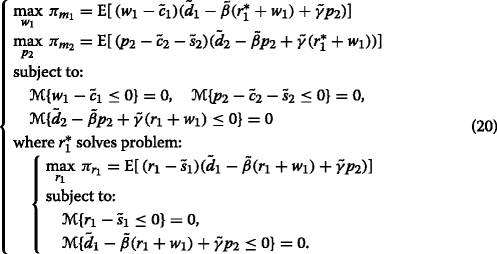Thus, for the given wholesale price w 1 and the retail price p 2, the optimal response of R1 in the decentralized chain should be derived firstly. Given (w 1,p 2), the profit function of R 1 is as follows:

\begin{aligned} \pi_{r_{1}}&=E[\tilde{d}_{1}]r_{1}-E[\tilde{\beta}] {r_{1}^{2}}-E[\tilde{\beta}] w_{1}r_{1}+E[\tilde{\gamma}] p_{2} r_{1}+ E[\tilde{\beta}^{1-\alpha}\tilde{s}_{1}^{1-\alpha}]r_{1}-E[\tilde{s}_{1}^{1-\alpha} \tilde{d}_{1}^{\alpha}]\\[0.3cm] &\qquad+ E[\tilde{\beta}^{1-\alpha}\tilde{s}_{1}^{1-\alpha}]w_{1}- E[\tilde{\gamma}^{\alpha}\tilde{s}_{1}^{1-\alpha}]p_{2}. \end{aligned}
(17)

Because the second-order derivative $$\frac {\partial ^{2}\pi _{r_{1}}(r_{1})}{\partial {r_{1}^{2}}}=-2E[\tilde {\beta }]<0$$, differentiating it and equating its first-order derivative to 0, we have

\begin{aligned} r_{1}^{*}(w_{1},p_{2})=\frac{E[\tilde{d}_{1}]+E[\tilde{\gamma}] p_{2}+E[\tilde{\beta}^{1-\alpha}\tilde{s}_{1}^{1-\alpha}]}{2E[\tilde{\beta}]}-\frac{1}{2}w_{1}. \end{aligned}
(18)

With the optimal response of the Stackelberg follower $$r_{1}^{*}(w_{1},p_{2})$$, the Nash-Stackelberg equilibrium of the two manufacturers can be obtained. Substituting $$r_{1}^{*}(w_{1},p_{2})$$ from Eq. (18) into the profit functions, we have

{} \begin{aligned} E[\pi_{m_{1}}]&=E\left[(w_{1}-\tilde{c}_{1})\left(\tilde{d}_{1}-\tilde{\beta}\left(\frac{E[\tilde{d}_{1}]+E[\tilde{\beta}^{1-\alpha}\tilde{s}_{1}^{1-\alpha}]+E[\tilde{\gamma} ]p_{2}}{2E[\tilde{\beta}]}+\frac{1}{2}w_{1}\right)+\tilde{\gamma}p_{2}\right)\right]\\ &=\frac{1}{2}\left\{E[\tilde{d}_{1}]w_{1}-E[\tilde{\beta}] {w_{1}^{2}}-E[\tilde{\beta}^{1-\alpha}\tilde{s}_{1}^{1-\alpha}]w_{1}+E[\tilde{\gamma}] p_{2}w_{1} -2E[\tilde{c}_{1}^{1-\alpha}\tilde{d}_{1}^{\alpha}]\right. \\ &\quad\!\!\left.\!+E\left[\!\tilde{c}_{1}^{1-\alpha}\tilde{\beta}^{1-\alpha}\!\right]\!w_{1}\!\,+\,E\left[\!\tilde{c}_{1}^{1-\alpha}\tilde{\beta}^{1-\alpha}\!\right]\!\frac{E[\!\tilde{d}_{1}] \,+\,E[\tilde{\beta}^{1-\alpha}\tilde{s}_{1}^{1-\alpha}]\,+\,E[\tilde{\gamma} ]p_{2}}{E[\tilde{\beta}]}\!\,-\,2E\left[\!\tilde{c_{1}}^{L}\tilde{\gamma}^{U}\!\right]p_{2}\right\},\\ E[\pi_{m_{2}}]&=E[(p_{2}-\tilde{c}_{2}-\tilde{s}_{2})\left(\tilde{d}_{2}-\tilde{\beta}p_{2}+\tilde{\gamma}\left(\frac{E[\tilde{d}_{1}]+E[\tilde{\beta}\tilde{s}_{1}]+E[\tilde{\gamma} ]p_{2}}{2E[\tilde{\beta}]}+\frac{1}{2}w_{1}\right)\right)]\\ &=E[\tilde{d}_{2}]p_{2}-\left(E[\tilde{\beta}]-\frac{E[\tilde{\gamma}]^{2}}{2E[\tilde{\beta}]}\right){p_{2}^{2}}+ \frac{E[\tilde{\gamma}]}{2E[\tilde{\beta}]}\left(E[\tilde{d}_{1}]+E[\tilde{s}_{1}\tilde{\beta}]\right)p_{2}+\frac{1}{2}E[\tilde{\gamma}]w_{1}p_{2}\\ &\quad-E\left[\tilde{c}_{2}^{1-\alpha}\tilde{d}_{2}^{\alpha}\right]-E\left[\tilde{s}_{2}^{1-\alpha}\tilde{d}_{2}^{\alpha}\right]+\left(E[\tilde{c}_{2}\tilde{\beta}]+E[\tilde{s}_{2}\tilde{\beta}]\right)p_{2}\\ &\quad-\left(E\left[\tilde{c}_{2}^{1-\alpha}\tilde{\gamma}^{\alpha}\right]+E\left[\tilde{s}_{2}^{1-\alpha}\tilde{\gamma}^{\alpha}\right]\right)\left(\frac{E[\tilde{d}_{1}]+E[\tilde{\beta}\tilde{s}_{1}]+E[\tilde{\gamma} ]p_{2}}{2E[\tilde{\beta}]}+\frac{1}{2}w_{1}\right). \end{aligned}
(19)

Differentiating these two functions, we have

\begin{aligned} \frac {\partial \pi_{m_{1}}(w_{1})}{\partial w_{1}}=&\frac{1}{2}\left(-2E[\tilde{\beta}] w_{1}+E[\tilde{\gamma}] p_{2}+E[\tilde{d}_{1}]+E\left[\tilde{c}_{1}^{1-\alpha}\tilde{\beta}^{1-\alpha}\right]-E\left[\tilde{s}_{1}^{1-\alpha}\tilde{\beta}^{1-\alpha}\right]\right),\\ \frac {\partial \pi_{m_{2}}(p_{2})}{\partial p_{2}}\,=\,&-\!2\left(E[\tilde{\beta}]-\frac{E[\tilde{\gamma}]^{2}}{2E[\tilde{\beta}]}\right)p_{2}\!\!+E[\tilde{d}_{2}]\ \!+\frac{E[\tilde{\gamma}]}{2E[\tilde{\beta}]}\!\left(E[\tilde{d}_{1}]\!+E[\tilde{s}_{1}\tilde{\beta}]\right) \!\!+\frac{1}{2}E[\tilde{\gamma}]w_{1}\\&\,+\,E\left[\tilde{c}_{2}^{1-\alpha}\tilde{\beta}^{1-\alpha}\right]\!+E\left[\tilde{s}_{2}^{1-\alpha}\tilde{\beta}^{1-\alpha}\right]\!-\frac{E[\tilde{\gamma} ]}{2E[\tilde{\beta}]}\left(E\left[\tilde{c}_{2}^{1-\alpha}\tilde{\gamma}^{\alpha}\right]\!+E\left[\tilde{s}_{2}^{1-\alpha}\tilde{\gamma}^{\alpha}\right]\right). \end{aligned}
(20)

With the assumption that $$E[\tilde {\beta }] >E[\tilde {\gamma }]>0$$, we have

$$\frac {\partial^{2}\pi_{m1}(w_{1})}{\partial {w_{1}^{2}}}=-E[\tilde{\beta}]<0,$$
$$\frac {\partial^{2}\pi_{m2}(p_{2})}{\partial {p_{2}^{2}}}=-2\left(E[\tilde{\beta}]-\frac{E[\tilde{\gamma}]^{2}}{2E[\tilde{\beta}]}\right)<-2E[\tilde{\beta}]+2\frac{E[\tilde{\beta}]^{2}}{2E[\tilde{\beta}]} =-E[\tilde{\beta}]<0.$$

Therefore, we can get the Nash equilibrium by setting the first-order derivative functions Eq. (20) equaling 0 as follows:

{} \begin{aligned} w_{1}^{*}=&\frac{E[\tilde{\gamma}]p_{2}^{*}+E[\tilde{d_{1}}]+E\left[\tilde{c_{1}}^{1-\alpha}\tilde{\beta}^{1-\alpha}\right] -E\left[\tilde{s_{1}}^{1-\alpha}\tilde{\beta}^{1-\alpha}\right]}{2E[\tilde{\beta}]},\\ p_{2}^{*}\!=&\frac{1}{8E[\tilde{\beta}]^{2}-5E[\tilde{\gamma}]^{2}}\left(\! E[\tilde{\gamma}](E\left[\tilde{c}_{1}^{1-\alpha}\tilde{\beta}^{1-\alpha}\!\right]\,+\,E\left[\tilde{s}_{1}^{1-\alpha}\tilde{\beta}^{1-\alpha}\right]\right) \,+\,3E[\tilde{\gamma}] E[\tilde{d}_{1}]\,+\,4E[\tilde{\beta}] E[\tilde{d}_{2}]\\ &\quad+4E[\tilde{\beta}]\left(E\left[\tilde{c}_{2}^{1-\alpha}\tilde{\beta}^{1-\alpha}\right]+E\left[\tilde{s}_{2}^{1-\alpha}\tilde{\beta}^{1-\alpha}\right]\right) -2E[\tilde{\gamma}] \left(E\left[\tilde{c}_{2}^{1-\alpha}\tilde{\gamma}^{\alpha}\right]+E\left[\tilde{s}_{2}^{1-\alpha}\tilde{\gamma}^{\alpha}\right]\right). \end{aligned}
(21)

### Pure Integrated Structures (II)

When both of the supply chains are vertically integrated, each distributes its product to consumers through its own channel directly, and then the competition between the two integrated manufacturers becomes a Nash (Bertrand) game.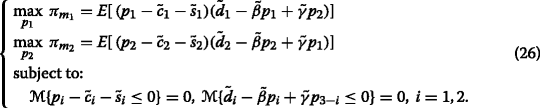Similar to the solution approach mentioned in the other models, we should get the crisp form of the uncertain objective functions first.

\begin{aligned} \pi_{m_{i}}=&-E[\tilde{\beta}] {p_{i}^{2}}+E[\tilde{\gamma}] p_{3-i}p_{i}+E[\tilde{d}_{i}]p_{i}+E[\tilde{\beta}^{1-\alpha}\tilde{c}_{i}^{1-\alpha}]p_{i}+E[\tilde{\beta}^{1-\alpha}\tilde{s}_{i}^{1-\alpha}] p_{i}\\ &-E[\tilde{c}_{i}^{1-\alpha}\tilde{d_{i}}^{\alpha}]-E[\tilde{s}_{i}^{1-\alpha}\tilde{d_{i}}^{\alpha}]-E[\tilde{c}_{i}^{1-\alpha}\tilde{\gamma}^{\alpha}]p_{3-i} +E[\tilde{s}_{i}^{1-\alpha}\tilde{\gamma}^{\alpha}] p_{3-i},\ i=1,2. \end{aligned}
(22)

From Eq. (22), we can get that the second-order derivatives of the objective functions $$\frac {\partial ^{2}\pi _{m_{i}}}{\partial {p_{i}^{2}}}=-2E[\tilde {\beta }]<0$$ with the assumption that $$E[\tilde {\beta }] >0$$. Therefore, $$\pi _{m_{i}}$$ are concave in p i , i=1,2. We can obtain the Nash equilibrium by setting the first-order derivatives equaling 0:

\begin{aligned} \frac {\partial\pi_{m_{1}}(p_{1},p_{2})}{\partial p_{1}}=-2E[\tilde{\beta}] p_{1}+E[\tilde{\gamma}] p_{2}+E[\tilde{d}_{1}]+E[\tilde{\beta}^{1-\alpha}\tilde{c}_{1}^{1-\alpha}]+E[\tilde{\beta}^{1-\alpha}\tilde{s}_{1}^{1-\alpha}]=0,\\ \frac {\partial\pi_{m_{2}}(p_{1},p_{2})}{\partial r_{1}}=-2E[\tilde{\beta}] p_{2}+E[\tilde{\gamma}] p_{1}+E[\tilde{d}_{2}]+E[\tilde{\beta}^{1-\alpha}\tilde{c}_{2}^{1-\alpha}]+E[\tilde{\beta}^{1-\alpha}\tilde{s}_{2}^{1-\alpha}]=0. \end{aligned}

The solution can be obtained as follows:

{} {\begin{aligned} p_{1}^{*}=\frac{2E[\tilde{\beta}](E[\tilde{d}_{1}]+E[\tilde{\beta}^{1-\alpha}\tilde{c}_{1}^{1-\alpha}]+E[\tilde{\beta}^{1-\alpha}\tilde{s}_{1}^{1-\alpha}])+E[\tilde{\gamma}] (E[\tilde{d}_{2}]+E[\tilde{\beta}^{1-\alpha}\tilde{c}_{2}^{1-\alpha}]+E[\tilde{\beta}^{1-\alpha}\tilde{s}_{2}^{1-\alpha}])}{4E[\tilde{\beta}]^{2}-E[\tilde{\gamma}]^{2}},\\ p_{2}^{*}=\frac{2E[\tilde{\beta}](E[\tilde{d}_{2}]+E[\tilde{\beta}^{1-\alpha}\tilde{c}_{2}^{1-\alpha}]+E[\tilde{\beta}^{1-\alpha}\tilde{s}_{2}^{1-\alpha}])+E[\tilde{\gamma}] (E[\tilde{d}_{1}]+E[\tilde{\beta}^{1-\alpha}\tilde{c}_{1}^{1-\alpha}]+E[\tilde{\beta}^{1-\alpha}\tilde{s}_{1}^{1-\alpha}])}{4E[\tilde{\beta}]^{2}-E[\tilde{\gamma}]^{2}}. \end{aligned}}

## Strategy Decision Analysis

Because of the complicated form of the equilibrium prices and expected profits, numerical examples rather than analytical comparisons are conducted to explore the effect of the uncertain degrees of the costs and demands on equilibrium prices. Without loss of generality of the conclusion, a series of experiments are conducted. As the results from different experiments are somewhat coherent, we present only one of them in this part.

Consider the case from Zhao et al.  that both the unit manufacturing costs are between 7 and 10, and most likely 8, the sales costs are between 2 and 5, and most likely 4, the market bases of the two products are both about 800, the elasticity coefficient $$\tilde {\beta }$$ is about 80 and $$\tilde {\gamma }$$ is about 50. These linguist estimations can be denoted as uncertain variables with distributions shown in Table 1.

According to Lemma 4, we have

{} \begin{aligned} E[\tilde{s}_{1}^{1-\alpha}\tilde{d_{1}}^{\alpha}]=&{\int_{0}^{1}}[\Phi_{s_{1}}^{-1}(1-\alpha)\Phi_{d_{1}}^{-1}(\alpha)]\mathrm{d}\alpha\\ = & \int_{0}^{0.5}[(700(1-\alpha)+900\alpha)((2-2(1-\alpha))*4+(2(1-\alpha)-1)*5]\mathrm{d}\alpha\\ &\ \ +\int_{0.5}^{1}(700(1-\alpha)+900\alpha)((1-2(1-\alpha))*2+(2(1-\alpha))*4)\mathrm{d}\alpha\\ =&\,2950 \end{aligned}
(23)

Similarly, we can get that the values of $$E[\tilde {c}_{1}^{1-\alpha }\tilde {\beta }^{1-\alpha }],E[\tilde {c}_{1}^{1-\alpha }\tilde {\gamma }^{\alpha }],E[\tilde {c}_{1}^{1-\alpha }\tilde {d_{1}}^{\alpha }], E[\tilde {s}_{1}^{1-\alpha }\tilde {\beta }^{1-\alpha }]$$ and $$E[\tilde {s}_{1}^{1-\alpha }\tilde {\gamma }^{\alpha }]$$ are 665.00,407.50,6550.00,305.00 and 182.50, respectively.

Note that $$E[\tilde {s}_{1}^{1-\alpha }\tilde {d_{1}}^{\alpha }]\not =E[\tilde {s}_{1}]E[\tilde {d_{1}}]$$. Different from independent random variables, the expected value of the product of two uncertain variables does not only depend on the expected value of each variable, but also on their distributions. Thus, the distributions of the uncertain parameters may have great influences on the pricing decisions. One may concern that how the uncertainty of the parameters affects the pricing decisions in operational level and the channel structure decisions in strategy level. The uncertainty or uncertain degree, defined by the distributions or ranges of uncertain variables which mainly depends on experts’ personal knowledge and also the accessibility and availability of the information concerning the uncertain parameters. More information about the parameters is available, more accurate estimations the experts can make, and consequently, distributions with smaller ranges can be attained and the uncertain degrees of the parameters will become lower. These results below can help the managers understand more about the pricing decisions with limited information and also help them make decisions in strategy level.

### Effects of Uncertain Degrees on Pricing Decisions

First of all, we analyze the effects of the uncertain degrees of price elastic coefficients $$\tilde {\beta },\tilde {\gamma }$$, the manufacturing costs $$\tilde {c_{1}}, \tilde {c_{2}}$$ and sales costs $$\tilde {s_{1}}, \tilde {s_{2}}$$ on optimal pricing decisions under the three possible structures. By varying the uncertain degrees of these parameters and keeping the other parameters unchanged, the changes of optimal prices are shown in Tables 2, 3, 4, 5, 6, and 7.

### Remark 1

The uncertain variable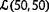degenerates to a real number when a=b. A real number is a special uncertain variable.

Referring to Table 2, we can find when the uncertain degree of parameter $$\tilde {\beta }$$ increases, the markup prices and retail prices will increase slightly while the wholesale prices will drop slightly in the pure decentralized structure. The wholesale price, markup price and retail price of the decentralized chain increase as well as the retail price of the integrated chain in the mixed structure. The retail prices of the pure integrated structure will decrease when the uncertain degree decreases.

Referring to Table 3, we find that the uncertain degree of the parameter $$\tilde {\gamma }$$ has no impact on the price decisions in the pure decentralized and integrated structures. The participants in the mixed structures, however, either in the decentralized chain or integrated chain, pursue higher prices when the uncertain degree increases.

Referring to Tables 4 and 5:

• The wholesale price, markup price and retail price will increase while the markup price of the retailer in the other chain will drop when the uncertain degree of the manufacturing cost increases in the pure decentralized structure.

• The retail price in the pure integrated structure as well as the price in the other chain will decrease when the uncertain degree of the manufacturing cost decreases.

• The manufacturer in the decentralized chain will choose a lower wholesale price, which in turn leads to a lower retail price when the uncertain degree of its manufacturing cost increases.

From Tables 6 and 7:

• The wholesale price, markup price, and retail price will increase when the uncertain degree of the sales cost increases while the wholesale price of the manufacturer in the other chain will drop in the pure decentralized structures.

• The wholesale price, markup price, and retail price in the mixed structure will drop slightly when the uncertain degree of the sales cost decreases, either in the decentralized chain or integrated chain.

• The sales price in the pure integrated structure will decrease with the decrease of the uncertain degree of the sales cost as well as the price in the other chain.

The other numerical examples show the same results with the above one. Similar to the numerical experiments above, more experiments can also be conducted to explore the impacts of uncertain degrees of the other parameters on the prices and profits of the two supply chains.

### Equilibrium Analysis of Structure Strategies

In this part, we explore what effects the uncertain degree of the market bases and competing intensity of the two products might have on the structure of the two competing supply chains.

In consideration of the equilibrium expected profits of the manufacturers at operational level under different structures and competing intensities, we can examine whether there is any economic incentive for a manufacturer to switch from integrated structure (I) to decentralized structure (D) in an industry where a pure decentralized structure is more profitable than a pure vertically integrated structure. A point which should not be neglected is that the expected profits are not comparably attained from different intensities, but we can only contrast the expected profits in different structures with the same competing intensities.

In the experiments, the price elasticity coefficients $$\tilde {\beta }$$ and $$\tilde {\gamma }$$ degenerate to crisp numbers, and the other data keep the same with the above experiments. Let β remain unchanged as 80 and vary γ from 0 to 80, i.e., the competing intensity $$\theta =\frac {\gamma }{\beta }$$ varies from 0 to 1. The results attained from the above three models in different scenarios are shown in Fig. 2.

From Fig. 2, the following results are obtained:

• If θθ 1, both the manufacturers will choose vertically integrated channel structure. Therefore, the two manufacturers can reach a Nash equilibrium in the strategy level by keeping vertically integrated.

• For θ 1<θ<θ 2, even though a pure decentralized structure can make the two manufacturers more profitable, the equilibrium of the two manufacturers is to keep vertically integrated. Given that both are decentralized, there is an economic incentive for the manufacturer moving to a vertically integrated structure. Thus, if the structure is hybrid, there is also an economic incentive for the integrated manufacturer selling through a company store. In other words, when the competing intensity is between (θ 1,θ 2), the two manufacturers may somewhat fall into a prisoner’s dilemma.

• Consequently, for θθ 2, the unique equilibrium of the two manufacturers is to keep decentralized ignoring the competitor’s structure.

The results have very intuitive highlight that if the products are highly competitive (the case that the two products are highly substitutable θ>θ 2), the manufacturers in duopoly supply chains are better off to choose an independent retailer to distribute their products rather than through company stores even though they can do it as efficiently as the privately-owned. This is in line with equilibriums concluded in McGuire and Staelin  in deterministic environment.

By the way, what we care most is the effect of the uncertain parameters on the equilibrium behaviors. In order to explore how the uncertain degrees impact the equilibrium behavior, another experiment is provided to make a contrast between the uncertain environment and deterministic environment. We examine the influence of the uncertainty on the equilibrium behaviors of the two manufacturers, and the result is presented in Fig. 3.

Referring to Fig. 3, we can find that $$\theta _{2}^{Uncertain}>\theta _{2}^{Deteministic}$$, $$\theta _{1}^{Uncertain}>\theta _{1}^{Deteministic}$$, illustrating that both in uncertain environment and deterministic environment, the duopoly manufacturers are better off to distribute their products through a decentralized channel rather than an integrated one when the competing intensity is higher than some value. Furthermore, uncertain environment will make the value higher than the one in deterministic environment.

## Conclusions

In this paper, we considered a pricing competing problem with two competing supply chains, each of which consists of a manufacturer and a single exclusive retailer and distributes differentiated but competing products in the same market. The manufacturing costs, sales costs, and demands were characterized as uncertain variables which is more in line with the real-life problem. Meanwhile, uncertainty theory and game-theory-based models were employed to formulate the pricing decision problems. The equilibrium behaviors of the participants at the operational level under three possible scenarios are derived from these models.

Numerical experiments were also given to explore the impact of uncertain degree of the parameters on the pricing decisions. The results demonstrated that these supply chain uncertain factors have great influences on the decision makers and sometimes they choose higher prices while sometimes they decide lower ones when the uncertain degree increases. In addition, We also illustrated the effects of competing intensity (substitutability) of the two products on the strategy behaviors in vertically integrated structure versus decentralized structure, of the manufacturers. It was found that the manufacturers are better off to distribute their products through a decentralized channel rather than an integrated one if the products are highly competitive. Specially, the uncertain environment will reduce the competition and increase some specific value of the competing intensity of the products, higher than which the duopoly manufacturers are better off to choose decentralized.

This paper focused on only one type of indeterminacy, while the real world might behave more complicated in which randomness and uncertainty might co-exist. Therefore, one possible extension of this paper is to study the pricing problem with twofold indeterminacy, in which uncertain random variable can be applied. In addition, this paper only considered the dealership structures, and future researches can be focused on some more complicated channel structures, containing multiple manufacturers and retailers. Besides, this paper assumed that all the participants are risk neutral while the decisionmakers may be risk sensitive in the real world. The research can be more applicable if the equilibrium behaviors with risk-sensitive members are considered.

## References

1. Liu, B: Uncertainty Theory. Springer, Berlin (2007).

2. Liu, B: Uncertainty Theory. Springer, Berlin (2010).

3. McGuire, TW, Staelin, R: An industry equilibrium analysis of downstream vertical integration. Mark. Sci. 2(2), 161–191 (1983).

4. Coughlan, AT: Competition and cooperation in marketing channel choice: theory and application. Mark. Sci. 4(2), 110–129 (1985).

5. Anderson, EJ, Bao, Y: Price competition with integrated and decentralized supply chains. Eur. J. Oper. Res. 200(1), 227–234 (2010).

6. Li, X, Li, Y: Chain-to-chain competition on product sustainability. J. Cleaner Prod. 112, 2058–2065 (2014).

7. Xiao, T, Yang, D: Price and service competition of supply chains with risk-averse retailers under demand uncertainty. Int. J. Prod. Econ. 114(1), 187–200 (2008).

8. Wu, D, Baron, O, Berman, O: Bargaining in competing supply chains with uncertainty. Eur. J. Oper. Res. 197(2), 548–556 (2009).

9. Shi, R, Zhang, J, Ru, J: Impacts of power structure on supply chains with uncertain demand. Prod. Oper. Manag. 22(5), 1232–1249 (2013).

10. Mahmoodi, A, Eshghi, K: Price competition in duopoly supply chains with stochastic demand. J. Manuf. Syst. 33(4), 604–612 (2014).

11. Zhou, C, Zhao, R, Tang, W: Two-echelon supply chain games in a fuzzy environment. Comput. Ind. Eng. 55(2), 390–405 (2008).

12. Zhao, J, Tang, W, Wei, J: Pricing decision for substitutable products with retail competition in a fuzzy environment. Int. J. Prod. Econ. 135(1), 144–153 (2012).

13. Zhao, J, Tang, W, Zhao, R, Wei, J: Pricing decisions for substitutable products with a common retailer in fuzzy environments. Eur. J. Oper. Res. 216(2), 409–419 (2012).

14. Zhao, J, Liu, W, Wei, J: Competition under manufacturer service and price in fuzzy environments. Knowledge-Based Syst. 50, 121–133 (2013).

15. Liu, S, Xu, Z: Stackelberg game models between two competitive retailers in fuzzy decision environment. Fuzzy Optimization Decis. Mak. 13(1), 33–48 (2014).

16. Ke, H, Huang, H, Ralescu, DA, Wang, L: Fuzzy bilevel programming with multiple non-cooperative followers: model, algorithm and application. Int. J. General Syst. 45(3), 336–351 (2016).

17. Yao, K, Ke, H: Entropy operator for membership function of uncertain set. Appl. Math. Comput. 242, 898–906 (2014).

18. Liu, H, Ke, H, Fei, W: Almost sure stability for uncertain differential equation. Fuzzy Optimization Decis. Mak. 13(4), 463–473 (2014).

19. Chen, X, Ning, Y, Wang, X: Convergence of complex uncertain sequences. J. Intell. Fuzzy Syst. 30(6), 3357–3366 (2014).

20. Chen, X: American option pricing formula for uncertain financial market. Int. J. Oper. Res. 8(2), 32–37 (2011).

21. Chen, X: Variation analysis of uncertain stationary independent increment processes. Eur. J. Oper. Res. 222(2), 312–316 (2012).

22. Bhattacharyya, R, Chatterjee, A, Kar, S: Uncertainty theory based multiple objective mean-entropy-skewness stock portfolio selection model with transaction costs. J. Uncertainty Anal. Appl. 1(1), 16 (2013).

23. Gao, Y: Uncertain models for single facility location problems on networks. Appl. Math. Model. 36(6), 2592–2599 (2012).

24. Yang, X, Gao, J: Uncertain differential games with application to capitalism. J. Uncertainty Anal. Appl. 1(1), 17 (2013).

25. Ke, H: A genetic algorithm-based optimizing approach for project time-cost trade-off with uncertain measure. J. Uncertainty Anal. Appl. 2(1), 8 (2014).

26. Ke, H: Uncertain random time-cost trade-off problem. J. Uncertainty Anal. Appl. 2, 23 (2014).

27. Ke, H, Liu, H, Tian, G: An uncertain random programming model for project scheduling problem. Int. J. Intell. Syst. 30(1), 66–79 (2015).

28. Wang, L, Huang, H, Ke, H: Chance-constrained model for rcpsp with uncertain durations. J. Uncertainty Anal. Appl. 3(1), 1 (2015).

29. Ma, W, Che, Y, Huang, H, Ke, H: Resource-constrained project scheduling problem with uncertain durations and renewable resources. Int. J. Mach. Learn. Cybernet. 7(4), 613–621 (2016).

30. Ke, H, Li, Y, Huang, H: Uncertain pricing decision problem in closed-loop supply chain with risk-averse retailer. J. Uncertainty Anal. Appl. 3(1), 1 (2015).

31. Chen, L, Peng, J, Liu, Z, Zhao, R: Pricing and effort decisions for a supply chain with uncertain information. Int. J. Prod. Res. (2016). doi:http://dx.doi.org/10.1080/00207543.2016.1204475.

32. Han, S, Peng, Z, Wang, S: The maximum flow problem of uncertain network. Inform. Sci. 265, 167–175 (2014).

33. Huang, H, Ke, H: Pricing decision problem for substitutable products based on uncertainty theory. J. Intell. Manuf. (2014). doi:http://dx.doi.org/10.1007/s10845-014-0991-7.

34. Liu, B: Some research problems in uncertainty theory. J. Uncertain Syst. 3(1), 3–10 (2009).

35. Liu, Y, Ha, M: Expected value of function of uncertain variables. J. Uncertain Syst. 4(3), 181–186 (2010).

36. Liu, B: Theory and practice of uncertain programming. Springer, Berlin (2009).

37. Jeuland, AP, Shugan, SM: Note-channel of distribution profits when channel members form conjectures. Mark. Sci. 7(2), 202–210 (1988).

38. Choi, SC: Price competition in a channel structure with a common retailer. Mark. Sci. 10(4), 271–296 (1991).

### Funding

This work was supported by the National Natural Science Foundation of China (No.71371141) and the Fundamental Research Funds for the Central Universities.

### Authors’ contributions

HH and HK carried out the study in the paper and drafted the first version of the manuscript. They designed the framework and with YC performed the analysis together. All authors read and approved the final manuscript.

### Competing interests

The authors declare that they have no competing interests.

## Author information

Authors

### Corresponding author

Correspondence to Hua Ke.

## Rights and permissions